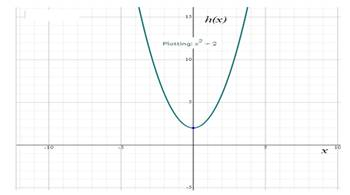# To write the four ways of representation method for the given data.### Precalculus: Mathematics for Calcu...

6th Edition
Stewart + 5 others
Publisher: Cengage Learning
ISBN: 9780840068071### Precalculus: Mathematics for Calcu...

6th Edition
Stewart + 5 others
Publisher: Cengage Learning
ISBN: 9780840068071

#### Solutions

Chapter 2.1, Problem 85E
To determine

## To write the four ways of representation method for the given data.

Expert Solution

The required table as shown below:

xh(x)=x2+22(2)2+2=61(1)2+2=30(0)2+2=21(1)2+2=3

Graph of the function as shown below:### Explanation of Solution

Given information: The function notation for the given description: h(x)=x2+2

The required function in words is h(x) equal to square the given variable x and add 2 to the result. The function h(x) is equal to square the given variable x and add 2 to the result.

Evaluate the values of the function h(x)=x2+2 for the estimate values of the variable x

Plug the values x=2,1,0,1 in the function h(x)=x2+2 then we get the values of h(x).

The required table as shown below:

xh(x)=x2+22(2)2+2=61(1)2+2=30(0)2+2=21(1)2+2=3

Graph of the function as shown below:### Have a homework question?

Subscribe to bartleby learn! Ask subject matter experts 30 homework questions each month. Plus, you’ll have access to millions of step-by-step textbook answers!